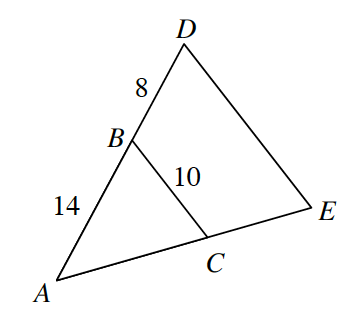### Home > INT2 > Chapter 7 > Lesson 7.2.2 > Problem7-85

7-85.In the diagram at right, $ΔABC$ ~ $ΔADE$.

1. Draw each triangle separately on your paper. Be sure to include all measurements in your diagrams.

1. Calculate the length of $\overline{DE}$.

Set up a proportion to solve for $DE$.

$DE\approx15.71$